# SIMPLE_BOUNDARY_CONDITION

Specifies element and nodal boundary conditions on a set of element faces.

AcuSolve Command

## Syntax

SIMPLE_BOUNDARY_CONDITION("name") {parameters...}

User-given name.

## Parameters

shape (enumerated) [no default]
Shape of the surfaces in this set.
three_node_triangle or tri3
Three-node triangle.
six_node_triangle or tri6
Six-node triangle.
element_set or elem_set (string) [no default]
User-given name of the parent element set.
surfaces (array) [no default]
List of element surfaces.
surface_sets (list) [={}]
List of surface set names (strings) to use in this simple boundary condition. When using this option, the connectivity, shape, and parent element of the surfaces are provided by the surface set container and it is unnecessary to specify the shape, element_set and surfaces parameters directly to the SIMPLE_BOUNDARY_CONDITION command. This option is used in place of directly specifying these parameters. In the event that both of the surface_sets and surfaces parameters are provided, the full collection of surface elements is read and a warning message is issued. The surface_sets option is the preferred method to specify the surface elements. This option provides support for mixed element topologies and simplifies pre-processing and post-processing.
type (enumerated) [=wall]
Type of the boundary surface.
wall
No-slip solid wall.
auto_wall
Automatic wall treatment.
inflow
Inflow boundary.
outflow
Outflow boundary.
slip
Slip surface.
symmetry
Plane of symmetry.
far_field
Either inflow or outflow depending on specified velocity.
free_surface
Free surface.
inflow_type (enumerated) [=velocity]
Type of the inflow boundary conditions. Used with inflow type.
velocity or vel
Nodal velocity and scalars.
pressure or pres
Weak pressure and nodal scalars.
stagnation_pressure or stag_pres
Weak stagnation pressure and nodal scalars.
mass_flux or mass
Integrated mass flux (equivalent to mass flow rate) and nodal scalars (except eddy viscosity).
flow_rate or flow
Integrated volume flux (equivalent to volumetric flow rate) and nodal scalars (except eddy viscosity).
average_velocity or ave_vel
Average velocity and nodal scalars (except eddy viscosity).
gravity_wave or wave
Nodal velocity, field and scalars for wave generator.
atmospheric
Atmospheric boundary layer.
velocity_pressure_temperature
All three variables (velocity, pressure, temperature) needed to specify supersonic inflow boundary condition. Used with compressible_navier_stokes equation.
mach_pressure_temperature
Another choice of the supersonic inflow boundary condition is Mach, pressure, and temperature. Used with compressible_navier_stokes equation.
humidity_type (enumerated) [=relative_humidity]
Type of humidity specified for the boundary condition. Used with inflow_type for the humid-air model.
relative_humidity
dewpoint_temperature
mass_fraction
relative_humidity (real) [=0]
Value of the relative humidity in percentage basis.
dewpoint_temperature (real) [=0]
Value of the dewpoint_temperature.
mass_fraction (list)[ ]
When humidity_type = mass_fration, field_boundary_conditions are used to specify mass fraction values of two fields.
outflow_type (enumerated) [=auto_pressure]
Type of the outflow boundary conditions. Used with outflow type.
auto_pressure
The user specified pressure is used in subsonic flow, while the user specified pressure is ignored if it is lower than the computed pressure on the outflow surface.
fix_pressure
Pressure is specified by you.
free_pressure
The user specified pressure is ignored.
back_flow_conditions (boolean) [=off]
Flag specifying whether to specify nodal boundary conditions for scalar variables on nodes on outflow surfaces where there is flow into the fluid domain. Used with outflow and far_field types.
temperature_back_flow_type or temp_back (enumerated) [=value]
How the constant temperature boundary condition is defined under back flow conditions. Used with outflow and far_field types with back_flow_conditions=on.
value
Specified value. Requires temperature.
area_average or area_ave
Average of solution over entire outflow surface.
exiting_area_average or exit_area_ave
Area average of solution over subset of outflow surface that has flow exiting the domain.
bulk
Bulk average of solution over entire outflow surface.
exiting_bulk or exit_bulk
Bulk average of solution over subset of outflow surface that has flow exiting the domain.
species_1_back_flow_type or species_1_back (enumerated) [=value]
How the constant species_1 boundary condition is defined under back flow conditions. Used with outflow and far_field types with back_flow_conditions=on.
value
Specified value. Requires species_1.
area_average or area_ave
Average of solution over entire outflow surface.
exiting_area_average or exit_area_ave
Area average of solution over subset of outflow surface that has flow exiting the domain.
mass_flux_average
Mass flux average of solution over entire outflow surface.
exiting_mass_flux_average
Mass flux average of solution over subset of outflow surface that has flow exiting the domain.
species_2_back_flow_type or species_2_back (enumerated) [=value]
How the constant species_2 boundary condition is defined under back flow conditions. Used with outflow and far_field types with back_flow_conditions=on.
value
Specified value. Requires species_s.
area_average or area_ave
Average of solution over entire outflow surface.
exiting_area_average or exit_area_ave
Area average of solution over subset of outflow surface that has flow exiting the domain.
mass_flux_average
Mass flux average of solution over entire outflow surface.
exiting_mass_flux_average
Mass flux average of solution over subset of outflow surface that has flow exiting the domain.
species_3_back_flow_type or species_3_back (enumerated) [=value]
How the constant species_3 boundary condition is defined under back flow conditions. Used with outflow and far_field types with back_flow_conditions=on.
value
Specified value. Requires species_3.
area_average or area_ave
Average of solution over entire outflow surface.
exiting_area_average or exit_area_ave
Area average of solution over subset of outflow surface that has flow exiting the domain.
mass_flux_average
Mass flux average of solution over entire outflow surface.
exiting_mass_flux_average
Mass flux average of solution over subset of outflow surface that has flow exiting the domain.
species_4_back_flow_type or species_4_back (enumerated) [=value]
How the constant species_4 boundary condition is defined under back flow conditions. Used with outflow and far_field types with back_flow_conditions=on.
value
Specified value. Requires species_4.
area_average or area_ave
Average of solution over entire outflow surface.
exiting_area_average or exit_area_ave
Area average of solution over subset of outflow surface that has flow exiting the domain.
mass_flux_average
Mass flux average of solution over entire outflow surface.
exiting_mass_flux_average
Mass flux average of solution over subset of outflow surface that has flow exiting the domain.
species_5_back_flow_type or species_5_back (enumerated) [=value]
How the constant species_5 boundary condition is defined under back flow conditions. Used with outflow and far_field types with back_flow_conditions=on.
value
Specified value. Requires species_5.
area_average or area_ave
Average of solution over entire outflow surface.
exiting_area_average or exit_area_ave
Area average of solution over subset of outflow surface that has flow exiting the domain.
mass_flux_average
Mass flux average of solution over entire outflow surface.
exiting_mass_flux_average
Mass flux average of solution over subset of outflow surface that has flow exiting the domain.
species_6_back_flow_type or species_6_back (enumerated) [=value]
How the constant species_6 boundary condition is defined under back flow conditions. Used with outflow and far_field types with back_flow_conditions=on.
value
Specified value. Requires species_6.
area_average or area_ave
Average of solution over entire outflow surface.
exiting_area_average or exit_area_ave
Area average of solution over subset of outflow surface that has flow exiting the domain.
mass_flux_average
Mass flux average of solution over entire outflow surface.
exiting_mass_flux_average
Mass flux average of solution over subset of outflow surface that has flow exiting the domain.
species_7_back_flow_type or species_7_back (enumerated) [=value]
How the constant species_7 boundary condition is defined under back flow conditions. Used with outflow and far_field types with back_flow_conditions=on.
value
Specified value. Requires species_7.
area_average or area_ave
Average of solution over entire outflow surface.
exiting_area_average or exit_area_ave
Area average of solution over subset of outflow surface that has flow exiting the domain.
mass_flux_average
Mass flux average of solution over entire outflow surface.
exiting_mass_flux_average
Mass flux average of solution over subset of outflow surface that has flow exiting the domain.
species_8_back_flow_type or species_8_back (enumerated) [=value]
How the constant species_8 boundary condition is defined under back flow conditions. Used with outflow and far_field types with back_flow_conditions=on.
value
Specified value. Requires species_8.
area_average or area_ave
Average of solution over entire outflow surface.
exiting_area_average or exit_area_ave
Area average of solution over subset of outflow surface that has flow exiting the domain.
mass_flux_average
Mass flux average of solution over entire outflow surface.
exiting_mass_flux_average
Mass flux average of solution over subset of outflow surface that has flow exiting the domain.
species_9_back_flow_type or species_9_back (enumerated) [=value]
How the constant species_9 boundary condition is defined under back flow conditions. Used with outflow and far_field types with back_flow_conditions=on.
value
Specified value. Requires species_9.
area_average or area_ave
Average of solution over entire outflow surface.
exiting_area_average or exit_area_ave
Area average of solution over subset of outflow surface that has flow exiting the domain.
mass_flux_average
Mass flux average of solution over entire outflow surface.
exiting_mass_flux_average
Mass flux average of solution over subset of outflow surface that has flow exiting the domain.
field_back_flow_type or field_back (enumerated) [=value]
How the constant field boundary condition is defined under back flow conditions. Used with outflow and far_field types with back_flow_conditions=on.
value
Specified value. Requires field_boundary_conditions.
field_boundary_conditions (list) [no default]
List of field boundary conditions that are applied at this boundary. Only used for type inflow and outflow. For inflow type, the values are applied to the fields directly. For outflow type, they are applied only if back_flow_conditions=on on the nodes where the flow is inbound.
viscoelastic_xx_back_flow_type or xxvest_back (enumerated) [=value]
How the constant viscoelastic_xx_stress boundary condition is defined under back flow conditions. Used with outflow and far_field types with back_flow_conditions=on.
value
Specified value. Requires viscoelastic_xx_stress.
area_average or area_ave
Average of solution over entire outflow surface.
exiting_area_average or exit_area_ave
Area average of solution over subset of outflow surface that has flow exiting the domain.
mass_flux_average
Mass flux average of solution over entire outflow surface.
exiting_mass_flux_average
Mass flux average of solution over subset of outflow surface that has flow exiting the domain.
viscoelastic_yy_back_flow_type or yyvest_back (enumerated) [=value]
How the constant viscoelastic_yy_stress boundary condition is defined under back flow conditions. Used with outflow and far_field types with back_flow_conditions=on.
value
Specified value. Requires viscoelastic_yy_stress.
area_average or area_ave
Average of solution over entire outflow surface.
exiting_area_average or exit_area_ave
Area average of solution over subset of outflow surface that has flow exiting the domain.
mass_flux_average
Mass flux average of solution over entire outflow surface.
exiting_mass_flux_average
Mass flux average of solution over subset of outflow surface that has flow exiting the domain.
viscoelastic_zz_back_flow_type or zzvest_back (enumerated) [=value]
How the constant viscoelastic_zz_stress boundary condition is defined under back flow conditions. Used with outflow and far_field types with back_flow_conditions=on.
value
Specified value. Requires viscoelastic_zz_stress.
area_average or area_ave
Average of solution over entire outflow surface.
exiting_area_average or exit_area_ave
Area average of solution over subset of outflow surface that has flow exiting the domain.
mass_flux_average
Mass flux average of solution over entire outflow surface.
exiting_mass_flux_average
Mass flux average of solution over subset of outflow surface that has flow exiting the domain.
viscoelastic_xy_back_flow_type or xyvest_back (enumerated) [=value]
How the constant viscoelastic_xy_stress boundary condition is defined under back flow conditions. Used with outflow and far_field types with back_flow_conditions=on.
value
Specified value. Requires viscoelastic_xy_stress.
area_average or area_ave
Average of solution over entire outflow surface.
exiting_area_average or exit_area_ave
Area average of solution over subset of outflow surface that has flow exiting the domain.
mass_flux_average
Mass flux average of solution over entire outflow surface.
exiting_mass_flux_average
Mass flux average of solution over subset of outflow surface that has flow exiting the domain.
viscoelastic_yz_back_flow_type or yzvest_back (enumerated) [=value]
How the constant viscoelastic_yz_stress boundary condition is defined under back flow conditions. Used with outflow and far_field types with back_flow_conditions=on.
value
Specified value. Requires viscoelastic_yz_stress.
area_average or area_ave
Average of solution over entire outflow surface.
exiting_area_average or exit_area_ave
Area average of solution over subset of outflow surface that has flow exiting the domain.
mass_flux_average
Mass flux average of solution over entire outflow surface.
exiting_mass_flux_average
Mass flux average of solution over subset of outflow surface that has flow exiting the domain.
viscoelastic_zx_back_flow_type or zxvest_back (enumerated) [=value]
How the constant viscoelastic_zx_stress boundary condition is defined under back flow conditions. Used with outflow and far_field types with back_flow_conditions=on.
value
Specified value. Requires viscoelastic_zx_stress.
area_average or area_ave
Average of solution over entire outflow surface.
exiting_area_average or exit_area_ave
Area average of solution over subset of outflow surface that has flow exiting the domain.
mass_flux_average
Mass flux average of solution over entire outflow surface.
exiting_mass_flux_average
Mass flux average of solution over subset of outflow surface that has flow exiting the domain.
eddy_viscosity_back_flow_type or eddy_back (enumerated) [=value]
How the constant eddy_viscosity boundary condition is defined under back flow conditions. Used with outflow and far_field types with back_flow_conditions=on.
value
Specified value. Requires eddy_viscosity.
area_average or area_ave
Average of solution over entire outflow surface.
exiting_area_average or exit_area_ave
Area average of solution over subset of outflow surface that has flow exiting the domain.
mass_flux_average
Mass flux average of solution over entire outflow surface.
exiting_mass_flux_average
Mass flux average of solution over subset of outflow surface that has flow exiting the domain.
kinetic_energy_back_flow_type or tke_back (enumerated) [=value]
How the constant turbulence_kinetic_energy boundary condition is defined under back flow conditions. Used with outflow and far_field types with back_flow_conditions=on.
value
Specified value. Requires kinetic_energy.
area_average or area_ave
Average of solution over entire outflow surface.
exiting_area_average or exit_area_ave
Area average of solution over subset of outflow surface that has flow exiting the domain.
mass_flux_average
Mass flux average of solution over entire outflow surface.
exiting_mass_flux_average
Mass flux average of solution over subset of outflow surface that has flow exiting the domain.
eddy_frequency_back_flow_type or tomega_back (enumerated) [=value]
How the constant turbulence eddy_frequency boundary condition is defined under back flow conditions. Used with outflow and far_field types with back_flow_conditions=on.
value
Specified value. Requires eddy_frequency.
area_average or area_ave
Average of solution over entire outflow surface.
exiting_area_average or exit_area_ave
Area average of solution over subset of outflow surface that has flow exiting the domain.
mass_flux_average
Mass flux average of solution over entire outflow surface.
exiting_mass_flux_average
Mass flux average of solution over subset of outflow surface that has flow exiting the domain.
dissipation_rate_back_flow_type or teps_back (enumerated) [=value]
How the constant turbulence dissipation_rate boundary condition is defined under back flow conditions. Used with outflow and far_field types with back_flow_conditions=on.
value
Specified value. Requires dissipation_rate.
area_average or area_ave
Average of solution over entire outflow surface.
exiting_area_average or exit_area_ave
Area average of solution over subset of outflow surface that has flow exiting the domain.
mass_flux_average
Mass flux average of solution over entire outflow surface.
exiting_mass_flux_average
Mass flux average of solution over subset of outflow surface that has flow exiting the domain.
intermittency_back_flow_type or tintc_back (enumerated) [=value]
How the constant turbulence transition intermittency boundary condition is defined under back flow conditions. Used with outflow and far_field types with back_flow_conditions=on.
value
Specified value. Requires intermittency.
area_average or area_ave
Average of solution over entire outflow surface.
exiting_area_average or exit_area_ave
Area average of solution over subset of outflow surface that has flow exiting the domain.
mass_flux_average
Mass flux average of solution over entire outflow surface.
exiting_mass_flux_average
Mass flux average of solution over subset of outflow surface that has flow exiting the domain.
transition_re_theta_back_flow_type or treth_back (enumerated) [=value]
How the constant turbulence dissipation_rate boundary condition is defined under back flow conditions. Used with outflow and far_field types with back_flow_conditions=on.
value
Specified value. Requires transition_re_theta.
area_average or area_ave
Average of solution over entire outflow surface.
exiting_area_average or exit_area_ave
Area average of solution over subset of outflow surface that has flow exiting the domain.
mass_flux_average
Mass flux average of solution over entire outflow surface.
exiting_mass_flux_average
Mass flux average of solution over subset of outflow surface that has flow exiting the domain.
precedence (integer) (=1)
Precedence of the nodal boundary conditions defined here with respect to others defined elsewhere. Highest value has precedence on a per-variable basis.
reference_frame (string) [=none]
User-given name of the reference frame for transforming velocity boundary conditions. If none, or if there are no velocity boundary conditions, no transformation takes place. Used with wall, inflow, slip, and symmetry types.
reference_frame_type (enumerated) [=direct]
Type of reference frame. Used with inflow type, wall type, auto_wall type and farfield type.
direct
Defined explicitly through the reference_frame identifier.
inherited
reference_frame is inherited from parent element set. Requires auto_wall type.
inflow_velocity_type (enumerated) [=cartesian]
Type of the inflow velocity. Used with inflow type and velocity inflow type.
cartesian
Velocity in cartesian coordinates. Requires x_velocity, y_velocity, and z_velocity.
cylindrical
Velocity in cylindrical coordinates. Requires cylinder_axis, axial_velocity, radial_velocity, and tangential_velocity.
spherical
Radial velocity component in spherical coordinates, other two components are zero. Requires sphere_center and radial_velocity.
normal
Velocity component normal to surface, other two components are zero. Requires normal_velocity.
waves list (={})
List of wave boundary conditions to generate waves at the inflow surface. Used with inflow type and gravity_wave inflow type.
heavy_fluid_velocity (array) (={0,0,0})
Velocity of the heavier fluid when used for a multiphase problem with two fluids and a stable interface. Used with inflow type and gravity_wave inflow_type.
light_fluid_velocity (array) (={0,0,0})
Velocity of the lighter fluid when used for a multiphase problem with two fluids and a stable interface. Used with inflow type and gravity_wave inflow type.
wave_damping (boolean) (=on)
Flag specifying whether to apply wave damping near the outflow surface. If off, no wave damping is applied near the outflow surface. Used with outflow type.
wall_velocity_type (enumerated) [=match_mesh_velocity]
Type of the wall velocity. Used with wall type.
zero
Velocity is zero.
match_mesh_velocity
Velocity matches velocity of the mesh.
cartesian
Velocity in cartesian coordinates. Requires x_velocity, y_velocity, and z_velocity.
cylindrical
Velocity in cylindrical coordinates. Requires cylinder_axis, axial_velocity, radial_velocity, and tangential_velocity.
spherical
Radial velocity component in spherical coordinates, other two components are zero. Requires sphere_center and radial_velocity.
normal
Velocity component normal to surface, other two components are zero. Requires normal_velocity.
cylinder_axis (array) [={0,0,0; 0,1,0}]
Coordinates of two points that define the axis of the cylinder. Used with inflow type and velocity inflow type and cylindrical inflow velocity type, or with wall type and cylindrical wall velocity type.
sphere_center (array) [={0,0,0}]
Coordinates of the center of the sphere. Used with inflow type and velocity inflow type and spherical inflow velocity type, or with wall type and spherical wall velocity type.
x_velocity or xvel (real) [=0]
Value of the x-velocity. Used with inflow type and velocity inflow type and cartesian inflow velocity type, or with wall type and cartesian wall velocity type.
x_velocity_multiplier_function (string) [=none]
User-given name of the multiplier function for scaling the x-velocity boundary condition value. If none, no scaling is performed. Used with x_velocity.
y_velocity or yvel (real) [=0]
Value of the y-velocity. Used with inflow type and velocity inflow type and cartesian inflow velocity type, or with wall type and cartesian wall velocity type.
y_velocity_multiplier_function (string) [=none]
User-given name of the multiplier function for scaling the y-velocity boundary condition value. If none, no scaling is performed. Used with y_velocity.
z_velocity or zvel (real) [=0]
Value of the z-velocity. Used with inflow type and velocity inflow type and cartesian inflow velocity type, or with wall type and cartesian wall velocity type.
z_velocity_multiplier_function (string) [=none]
User-given name of the multiplier function for scaling the z-velocity boundary condition value. If none, no scaling is performed. Used with z_velocity.
axial_velocity (real) [=0]
Value of the axial velocity. Used with inflow type and velocity inflow type and cylindrical inflow velocity type, or with wall type and cylindrical wall velocity type.
axial_velocity_multiplier_function (string) [=none]
User-given name of the multiplier function for scaling the axial velocity boundary condition value. If none, no scaling is performed. Used with axial_velocity.
Value of the radial velocity. Used with inflow type and velocity inflow type and cylindrical and spherical inflow velocity types, or with wall type and cylindrical and spherical wall velocity types.
User-given name of the multiplier function for scaling the radial velocity boundary condition value. If none, no scaling is performed. Used with radial_velocity.
tangential_velocity (real) [=0]
Value of the tangential velocity. Used with inflow type and velocity inflow type and cylindrical inflow velocity type, or with wall type and cylindrical wall velocity type.
tangential_velocity_multiplier_function (string) [=none]
User-given name of the multiplier function for scaling the tangential velocity boundary condition value. If none, no scaling is performed. Used with tangential_velocity.
normal_velocity (real) [=0]
Value of the normal velocity. Used with inflow type and velocity inflow type and normal inflow velocity type, or with wall type and normal wall velocity type.
normal_velocity_multiplier_function (string) [=none]
User-given name of the multiplier function for scaling the normal velocity boundary condition value. If none, no scaling is performed. Used with normal_velocity.
mass_flux or mass (real) >0 [=1]
Value of the integrated mass flux. Used with inflow type and mass_flux inflow type.
mass_flux_multiplier_function (string) [=none]
User-given name of the multiplier function for scaling the integrated mass flux value. If none, no scaling is performed. Used with inflow type and mass_flux inflow type.
flow_rate or flow (real) >0 [=1]
Value of the integrated flow rate. Used with inflow type and flow_rate inflow type.
flow_rate_multiplier_function (string) [=none]
User-given name of the multiplier function for scaling the integrated flow rate value. If none, no scaling is performed. Used with inflow type and flow_rate inflow type.
average_velocity or ave_vel (real) >0 [=1]
Value of the average velocity. Used with inflow type and average_velocity inflow type.
average_velocity_multiplier_function (string) [=none]
User-given name of the multiplier function for scaling the average velocity value. If none, no scaling is performed. Used with inflow type and average_velocity inflow type.
inflow_mach_number_type (enumerated) [=cartesian]
Type of the inflow mach number. Used with inflow type and mach_pressure_temperature type.
cartesian
Mach number in cartesian coordinates.
normal
Mach number component normal to surface, the other two components are zero.
mach_number (real) [=0]
Value of the Mach number. Used with inflow type and compressible_navier_stokes.
mach_number_multiplier_function (string) [=none]
User-given name of the multiplier function for scaling the mach number boundary condition value. If none, no scaling is performed. Used with mach_number.
mach_number_dir (array) [={1,0,0}]
Mach number direction. Used with inflow type, mach_pressure_temperature inflow_type and inflow_mach_number_type as well as far_field type.
pressure or pres (real) [=0]
Value of the pressure. Used with outflow, far_field, and free_surface types, and inflow type with pressure inflow type.
pressure_multiplier_function (string) [=none]
User-given name of the multiplier function for scaling the pressure boundary condition value. If none, no scaling is performed. Used with pressure.
pressure_loss_factor (real) >=0 [=0]
Coefficient of a pressure loss term added/subtracted to outflow/inflow pressure boundary conditions. Used with outflow, far_field, and free_surface types, and inflow type with pressure inflow type.
pressure_loss_factor_multiplier_function (string) [=none]
User-given name of the multiplier function for scaling the pressure loss factor. Used with outflow, far_field, and free_surface types, and inflow type with pressure inflow type. If none, no scaling is performed.
hydrostatic_pressure (boolean) [=off]
Flag specifying whether to add hydrostatic pressure to pressure and stagnation pressure boundary conditions. Used with outflow, far_field, and free_surface types, and inflow type with pressure and stagnation_pressure inflow types.
hydrostatic_pressure_origin (array) [={0,0,0}]
Coordinates of any location where the hydrostatic pressure is zero. Used with hydrostatic_pressure=on.
stagnation_pressure or stag_pres (real) [=0]
Value of the stagnation pressure. Used with inflow type and stagnation_pressure inflow type.
stagnation_pressure_multiplier_function (string) [=none]
User-given name of the multiplier function for scaling the stagnation pressure boundary condition value. If none, no scaling is performed. Used with stagnation_pressure.
temperature_type or temp_type (enumerated) [=flux]
Type of the thermal boundary condition. Used with wall type.
none
No thermal boundary condition.
value
Nodal boundary condition.
flux
Heat flux boundary condition.
temperature or temp (real) [=0]
Value of the temperature. Used with inflow type, and wall type with value temperature type. Also used with outflow and far_field types when back_flow_conditions = on.
temperature_multiplier_function (string) [=none]
User-given name of the multiplier function for scaling the temperature boundary condition value. If none, no scaling is performed. Used with temperature.
temperature_coupling_type (enumerated) [= tied]
Type of the temperature boundary condition used for thermal simulations for the nodes/elements of this SIMPLE_BOUNDARY_CONDITION command that match up with elements/nodes of a MESH_BOUNDARY_CONDITION of type=external_code. In the event that no matching MESH_BOUNDARY_CONDITION of type=external_code is found for the elements within this SIMPLE_BOUNDARY_CONDITION command, this parameter is ignored.
none
No temperature coupling with external code.
tied
Temperature coupling with external code.
heat_flux or heat (real) [=0]
Value of the heat flux. Used with wall type and flux temperature type.
heat_flux_multiplier_function (string) [=none]
User-given name of the multiplier function for scaling the heat flux boundary condition value. If none, no scaling is performed. Used with heat_flux.
convective_heat_coefficient (real) [=0]
Value of the convective heat coefficient. Used with wall type and flux temperature type.
convective_heat_multiplier_function (string) [=none]
User-given name of the multiplier function for scaling the convective heat coefficient value. If none, no scaling is performed. Used with convective_heat_coefficient.
convective_heat_reference_temperature (real) [=273.15]
Value of the reference temperature associated with the convective heat coefficient. Used with wall type and flux temperature type.
nucleate_boiling (boolean) [=off]
Flag specifying whether to activate single-phase nucleate boiling on walls. If off, single-phase nucleate-boiling is not applied. Used with wall type.
species_1_type or spec1_type (enumerated) [=flux]
Type of the species_1 boundary condition. Used with wall type.
value
Nodal boundary condition.
flux
Flux boundary condition.
species_1 or spec1 (real) [=0]
Value of the species_1. Used with inflow type, and wall type with value species_1 type. Also used with outflow and far_field types when back_flow_conditions = on.
species_1_multiplier_function (string) [=none]
User-given name of the multiplier function for scaling the species_1 boundary condition value. If none, no scaling is performed. Used with species_1.
species_1_flux (real) [=0]
Value of the species_1 flux. Used with wall type and species_1 type.
species_1_flux_multiplier_function (string) [=none]
User-given name of the multiplier function for scaling the species_1 flux boundary condition value. If none, no scaling is performed. Used with species_1_flux.
species_2_type or spec2_type (enumerated) [=flux]
Type of the species_2 boundary condition. Used with wall type.
value
Nodal boundary condition.
flux
Flux boundary condition.
species_2 or spec2 (real) [=0]
Value of the species_2. Used with inflow type, and wall type with value species_2 type. Also used with outflow and far_field types when back_flow_conditions = on.
species_2_multiplier_function (string) [=none]
User-given name of the multiplier function for scaling the species_2 boundary condition value. If none, no scaling is performed. Used with species_2.
species_2_flux (real) [=0]
Value of the species_2 flux. Used with wall type and flux species_2 type.
species_2_flux_multiplier_function (string) [=none]
User-given name of the multiplier function for scaling the species_2_flux boundary condition value. If none, no scaling is performed. Used with species_2_flux.
species_3_type or spec3_type (enumerated) [=flux]
Type of the species_3 boundary condition. Used with wall type.
value
Nodal boundary condition.
flux
Flux boundary condition.
species_3 or spec3 (real) [=0]
Value of the species_3. Used with inflow type, and wall type with value species_3 type. Also used with outflow and far_field types when back_flow_conditions = on.
species_3_multiplier_function (string) [=none]
User-given name of the multiplier function for scaling the species_3 boundary condition value. If none, no scaling is performed. Used with species_3.
species_3_flux (real) [=0]
Value of the species_3 flux. Used with wall type and flux species_3 type.
species_3_flux_multiplier_function (string) [=none]
User-given name of the multiplier function for scaling the species_3_flux boundary condition value. If none, no scaling is performed. Used with species_3_flux.
species_4_type or spec4_type (enumerated) [=flux]
Type of the species_4 boundary condition. Used with wall type.
value
Nodal boundary condition.
flux
Flux boundary condition.
species_4 or spec4 (real) [=0]
Value of the species_4. Used with inflow type, and wall type with value species_4 type. Also used with outflow and far_field types when back_flow_conditions = on.
species_4_multiplier_function (string) [=none]
User-given name of the multiplier function for scaling the species_4 boundary condition value. If none no scaling is performed. Used with species_4.
species_4_flux (real) [=0]
Value of the species_4 flux. Used with wall type and flux species_4 type.
species_4_flux_multiplier_function (string) [=none]
User-given name of the multiplier function for scaling the species_4_flux boundary condition value. If none, no scaling is performed. Used with species_4_flux.
species_5_type or spec5_type (enumerated) [=flux]
Type of the species_5 boundary condition. Used with wall type.
value
Nodal boundary condition.
flux
Flux boundary condition.
species_5 or spec5 (real) [=0]
Value of the species_5. Used with inflow type, and wall type with value species_5 type. Also used with outflow and far_field types when back_flow_conditions = on.
species_5_multiplier_function (string) [=none]
User-given name of the multiplier function for scaling the species_5 boundary condition value. If none, no scaling is performed. Used with species_5.
species_5_flux (real) [=0]
Value of the species_5 flux. Used with wall type and flux species_5 type.
species_5_flux_multiplier_function (string) [=none]
User-given name of the multiplier function for scaling the species_5 flux boundary condition value. If none, no scaling is performed. Used with species_5_flux.
species_6_type or spec6_type (enumerated) [=flux]
Type of the species_6 boundary condition. Used with wall type.
value
Nodal boundary condition.
flux
Flux boundary condition.
species_6 or spec6 (real) [=0]
Value of the species_6. Used with inflow type, and wall type with value species_6 type. Also used with outflow and far_field types when back_flow_conditions = on.
species_6_multiplier_function (string) [=none]
User-given name of the multiplier function for scaling the species_6 boundary condition value. If none, no scaling is performed. Used with species_6.
species_6_flux (real) [=0]
Value of the species_6 flux. Used with wall type and flux species_6 type.
species_6_flux_multiplier_function (string) [=none]
User-given name of the multiplier function for scaling the species_6 flux boundary condition value. If none, no scaling is performed. Used with species_6_flux.
species_7_type or spec7_type (enumerated) [=flux]
Type of the species_7 boundary condition. Used with wall type.
value
Nodal boundary condition.
flux
Flux boundary condition.
species_7 or spec7 (real) [=0]
Value of the species_7. Used with inflow type, and wall type with value species_7 type. Also used with outflow and far_field types when back_flow_conditions=on.
species_7_multiplier_function (string) [=none]
User-given name of the multiplier function for scaling the species_7 boundary condition value. If none, no scaling is performed. Used with species_7.
species_7_flux (real) [=0]
Value of the species_7 flux. Used with wall type and flux species_7 type.
species_7_flux_multiplier_function (string) [=none]
User-given name of the multiplier function for scaling the species_7 flux boundary condition value. If none, no scaling is performed. Used with species_7_flux.
species_8_type or spec8_type (enumerated) [=flux]
Type of the species_8 boundary condition. Used with wall type.
value
Nodal boundary condition.
flux
Flux boundary condition.
species_8 or spec8 (real) [=0]
Value of the species_8. Used with inflow type, and wall type with value species_8 type. Also used with outflow and far_field types when back_flow_conditions = on.
species_8_multiplier_function (string) [=none]
User-given name of the multiplier function for scaling the species_8 boundary condition value. If none no scaling is performed. Used with species_8.
species_8_flux (real) [=0]
Value of the species_8 flux. Used with wall type and flux species_8 type.
species_8_flux_multiplier_function (string) [=none]
User-given name of the multiplier function for scaling the species_8 flux boundary condition value. If none, no scaling is performed. Used with species_8_flux.
species_9_type or spec9_type (enumerated) [=flux]
Type of the species_9 boundary condition. Used with wall type.
value
Nodal boundary condition.
flux
Flux boundary condition.
species_9 or spec9 (real) [=0]
Value of the species_9. Used with inflow type, and wall type with value species_9 type. Also used with outflow and far_field types when back_flow_conditions = on.
species_9_multiplier_function (string) [=none]
User-given name of the multiplier function for scaling the species_9 boundary condition value. If none, no scaling is performed. Used with species_9.
species_9_flux (real) [=0]
Value of the species_9 flux. Used with wall type and flux species_9 type.
species_9_flux_multiplier_function (string) [=none]
User-given name of the multiplier function for scaling the species_9 flux boundary condition value. If none, no scaling is performed. Used with species_9_flux.
maintain_ambient_turbulence or amb_turb (boolean) [=off]
Flag specifying whether to maintain inflow turbulence intensity throughout the domain. Valid for all two-equation turbulence models. Used with inflow type, velocity inflow_type and farfield type.
synthetic_turbulence (boolean) [=off]
Flag specifying whether to activate the synthetic turbulence feature at inflow. Used with velocity, atmospheric inflow type. This command is only activated when synthetic_turbulence_input_type in turbulence_model_parameters is set to k_omega (or k_epsilon).
turbulence_input_type (enumerated) [=direct]
Type of turbulence input at inflow. Used with inflow type, with velocity, pressure, stagnation_pressure, inflow_type and farfield type.
direct
Direct specification of turbulence quantities. Requires associated turbulent value associated with selected turbulence model.
auto
Automatic definition for turbulence input. Valid for all turbulence models.
intensity_length_scale or tilen
Specifies turbulence quantities based on turbulence_intensity and length_scale. Valid for all turbulence models.
intensity_viscosity_ratio or tivr
Specifies turbulence quantities based on turbulence_intensity and viscosity_ratio. Valid for two-equation turbulence models.
viscosity_ratio or vr
Specifies turbulence quantities based on viscosity_ratio. Valid for the spalart_allmaras turbulence model.
turbulence_flow_type (enumerated) [=internal]
Type of turbulence flow type at inflow. Used with inflow type, with velocity, pressure, stagnation_pressure, inflow_type and farfield type.
internal
Specifies turbulent state at inflow for internal flows. Used with turbulence_input_type auto, intensity_length_scale and intensity_viscosity_ratio.
external
Specifies turbulent state at inflow for external flows. Used with turbulence_input_type auto, intensity_length_scale and intensity_viscosity_ratio.
turbulence_intensity_type (enumerated) [=auto]
Type of turbulence intensity at inflow. Used with inflow type and velocity, pressure, and stagnation_pressure inflow types. Also used with outflow and far_field types when back_flow_conditions = on.
auto
Automatic definition for turbulence intensity. Valid for all turbulence models.
value
User specified turbulence intensity at inflow.
low
Specifies turbulence intensity for low turbulence inflow simulations.
medium
Specifies turbulence intensity for medium turbulence inflow simulations.
high
Specifies turbulence intensity for high turbulence inflow simulations.
percent_turbulence_intensity (real) [=0.1]
Value of the turbulence intensity at the inflow. Used with inflow type and velocity, pressure, and stagnation_pressure inflow types. Also used with outflow and far_field types when back_flow_conditions = on.
turbulence_velocity_scale (real) [=0.0]
Value of the inflow velocity scale used to compute turbulent properties. Used with inflow type and velocity, pressure, and stagnation_pressure inflow types. Also used with outflow and far_field types when back_flow_conditions = on.
turbulence_length_scale (real) [=0.0]
Value of the inflow length scale used to compute turbulent properties. Used with inflow type and velocity, pressure, and stagnation_pressure inflow types. Also used with outflow and far_field types when back_flow_conditions = on.
turbulence_viscosity_ratio (real) [=0.1]
Value of the inflow turbulence viscosity ratio used to compute turbulent properties. Used with inflow type and velocity, pressure, and stagnation_pressure inflow types. Also used with outflow and far_field types when back_flow_conditions = on.
viscoelastic_xx_stress or xxvest [=0]
Value of the viscoelastic xx stress component. Used with inflow type and velocity, pressure, and stagnation_pressure inflow types. Also used with outflow and far_field types when back_flow_conditions = on.
viscoelastic_xx_stress_multiplier_function (string) [=none]
User-given name of the multiplier function for scaling the viscoelastic xx stress boundary condition value. If none, no scaling is performed. Used with viscoelastic_xx_stress.
viscoelastic_yy_stress or yyvest [=0]
Value of the viscoelastic yy stress component. Used with inflow type and velocity, pressure, and stagnation_pressure inflow types. Also used with outflow and far_field types when back_flow_conditions = on.
viscoelastic_yy_stress_multiplier_function (string) [=none]
User-given name of the multiplier function for scaling the viscoelastic yy stress boundary condition value. If none, no scaling is performed. Used with viscoelastic_yy_stress.
viscoelastic_zz_stress or zzvest [=0]
Value of the viscoelastic zz stress component. Used with inflow type and velocity, pressure, and stagnation_pressure inflow types. Also used with outflow and far_field types when back_flow_conditions = on.
viscoelastic_zz_stress_multiplier_function (string) [=none]
User-given name of the multiplier function for scaling the viscoelastic zz stress boundary condition value. If none, no scaling is performed. Used with viscoelastic_zz_stress.
viscoelastic_xy_stress or xyvest [=0]
Value of the viscoelastic xy stress component. Used with inflow type and velocity, pressure, and stagnation_pressure inflow types. Also used with outflow and far_field types when back_flow_conditions = on.
viscoelastic_xy_stress_multiplier_function (string) [=none]
User-given name of the multiplier function for scaling the viscoelastic xy stress boundary condition value. If none, no scaling is performed. Used with viscoelastic_xy_stress.
viscoelastic_yz_stress or yzvest [=0]
Value of the viscoelastic yz stress component. Used with inflow type and velocity, pressure, and stagnation_pressure inflow types. Also used with outflow and far_field types when back_flow_conditions = on.
viscoelastic_yz_stress_multiplier_function (string) [=none]
User-given name of the multiplier function for scaling the viscoelastic yz stress boundary condition value. If none, no scaling is performed. Used with viscoelastic_yz_stress.
viscoelastic_zx_stress or zxvest [=0]
Value of the viscoelastic zx stress component. Used with inflow type and velocity, pressure, and stagnation_pressure inflow types. Also used with outflow and far_field types when back_flow_conditions = on.
viscoelastic_zx_stress_multiplier_function (string) [=none]
User-given name of the multiplier function for scaling the viscoelastic zx stress boundary condition value. If none, no scaling is performed. Used with viscoelastic_zx_stress.
eddy_viscosity or eddy (real) [=0]
Value of the kinematic eddy viscosity. Used with inflow type and velocity, pressure, and stagnation_pressure inflow types. Also used with outflow and far_field types when back_flow_conditions = on.
eddy_viscosity_multiplier_function (string) [=none]
User-given name of the multiplier function for scaling the eddy viscosity boundary condition value. If none, no scaling is performed. Used with eddy_viscosity.
kinetic_energy or tke (real) [=0]
Value of the turbulent kinetic energy. Used with inflow type and velocity, pressure, and stagnation_pressure inflow types. Also used with outflow and far_field types when back_flow_conditions = on.
kinetic_energy_multiplier_function (string) [=none]
User-given name of the multiplier function for scaling the turbulent kinetic energy boundary condition value. If none, no scaling is performed. Used with kinetic_energy.
eddy_frequency or tomega (real) [=0]
Value of the turbulent eddy frequency. Used with inflow type and velocity, pressure, and stagnation_pressure inflow types. Also used with outflow and far_field types when back_flow_conditions = on.
eddy_frequency_multiplier_function (string) [=none]
User-given name of the multiplier function for scaling the turbulent eddy frequency boundary condition value. If none, no scaling is performed. Used with eddy_frequency.
dissipation_rate or teps (real) [=0]
Value of the turbulent dissipation rate. Used with inflow type and velocity, pressure, and stagnation_pressure inflow types. Also used with outflow and far_field types when back_flow_conditions = on.
dissipation_rate_multiplier_function (string) [=none]
User-given name of the multiplier function for scaling the turbulent dissipation rate boundary condition value. If none, no scaling is performed. Used with dissipation_rate.
turbulence_wall_type (enumerated) [=wall_function]
Type of the turbulence wall modeling. Used with wall type.
none
No wall modeling. When this option is set, the surface does not participate in the wall distance calculation for turbulence and no wall modeling is performed.
low_reynolds_number or low_re
Low Reynolds number damping functions.
wall_function or func
Turbulence wall function.
running_average_wall_function
Turbulence wall function based on running average variables.
roughness_height (real) >=0 [=0]
Average wall roughness height. Used with low_reynolds_number, wall_function, and running_average_wall_function turbulence wall types.
wall_function_heat_flux_factor >=1 [=1]
Constant factor used to scale the turbulent thermal conductivity within the first element off the wall. Used with turbulence_wall_type = wall_function and running_average_wall_function .
non_reflecting_factor (real) >=0 [=0]
Amount of non-reflecting modification. If zero, there is no effect. If one, waves can pass through the boundary without reflection. Used with pressure variable with outflow type and mass_flux variable with inflow type. Turning on both non_reflecting_bc_running_average_field and running_average specifies the use of running average fields to enhance the performance of non-reflective boundary conditions when flow = navier_stokes is used. When flow = compressible_navier_stokes is set, the running_average option does not need to be turned on.
surface_tension_model (string) [=none]
User-given name of the surface tension model. If none, surface tension is not modeled. Used with free_surface type. This parameter has been migrated to the MESH_BOUNDARY_CONDITION command.
contact_angle_model (string) [=none]
User-given name of the contact angle model. If none, the default contact angle model is used. Used with surface_tension_model and multi_field=levelset as well as wall_type.
mesh_displacement_type (enumerated) [=fixed]
Type of the wall for mesh displacement boundary conditions. Not used with free_surface type. This parameter has been migrated to the MESH_BOUNDARY_CONDITION command.
none
No mesh displacement boundary conditions.
fixed
Mesh fixed to the wall.
slip
Mesh slips tangentially along the wall.
flexible_body
Mesh follows a flexible body. Requires flexible_body.
guide_surface
Mesh follows a guide surface. Requires guide_surface.
flexible_body (string) [=none]
User-given name of the FLEXIBLE_BODY command. Used with flexible_body mesh displacement type. This parameter has been migrated to the MESH_BOUNDARY_CONDITION command.
nodal_x_modes (array) [no default]
Array of node number (first column) and x-component eigenvector values (last num_modes columns, where num_modes is given by the FLEXIBLE_BODY command referenced by flexible_body). Nodes must match those referenced in the surface. Used with flexible_body mesh displacement type. This parameter has been migrated to the MESH_BOUNDARY_CONDITION command.
nodal_y_modes (array) [no default]
Array of node number (first column) and y-component eigenvector values (last num_modes columns, where num_modes is given by the FLEXIBLE_BODY command referenced by flexible_body). Nodes must match those referenced in the surface. Used with flexible_body mesh displacement type. This parameter has been migrated to the MESH_BOUNDARY_CONDITION command.
nodal_z_modes (array) [no default]
Array of node number (first column) and z-component eigenvector values (last num_modes columns, where num_modes is given by the FLEXIBLE_BODY command referenced by flexible_body). Nodes must match those referenced in the surface. Used with flexible_body mesh displacement type. This parameter has been migrated to the MESH_BOUNDARY_CONDITION command.
guide_surface (string) [=none]
User-given name of the GUIDE_SURFACE command. Used with guide_surface mesh displacement type. This parameter has been migrated to the MESH_BOUNDARY_CONDITION command.
mesh_motion (string) [=none]
User-given name of the MESH_MOTION command for determining mesh displacement boundary conditions. If none, this parameter has no effect. Used with fixed and flexible_body mesh displacement types. This parameter has been migrated to the MESH_BOUNDARY_CONDITION command.
split_internal_surfaces (boolean) [=off]
Option to automatically split the nodes on internal surfaces that require two boundary conditions, that is, cases that include baffles, sliding mesh or thermal interfaces.
gap_factor (real) >=0 [=1]
Non-dimensional (with respect to the length of an element face) maximum gap allowed for two element faces to be in contact. No maximum if zero.
gap (real) >= 0 [=0]
Dimensional maximum gap allowed for two element faces to be in contact. No maximum if zero.
crease_angle or angle (real) >=0 <=180 [=90]
Maximum angle between face normals allowed for two element faces to be in contact. No maximum if zero.
active_type (enumerated) [=all]
Type of the active flag. Determines which surfaces in this set will have boundary conditions imposed by this command.
all
All surfaces in this set are active.
none
No surface in this set is active.
no_interface
Only surfaces that are not in an interface surface set or do not find a contact surface of an appropriate medium are active.
atmospheric_roughness_type (enumerated) (=suburb)
Type of the surface roughness for atmospheric boundary layer. Provides typical terrain types and user specified input.
user_value
User specification of roughness height.
ocean
Use roughness height for ocean (0.001m).
low_grass
Use roughness height for low grass (0.02m).
high_grass
Use roughness height for high grass (0.06m).
forest
Use roughness height for forest (1.0m).
suburb
Use roughness height for suburb (0.3m).
town
Use roughness height for town (1.0m).
city
Use roughness height for city (2.5m).
atmospheric_reference_velocity_type (enumerated) (=friction_velocity)
Type of the reference velocity for atmospheric boundary layer.
friction_velocity
Use ground friction velocity.
sample_height_velocity
Use height and corresponding velocity.
atmospheric_ground_roughness (real) (=0.001)
Value of roughness height if atmospheric_roughness_type = user_value.
atmospheric_friction_velocity (real) (=1.0)
Value of friction velocity if atmospheric_reference_velocity_type = friction_velocity.
atmospheric_sample_velocity (real) (=5.0)
Value of reference velocity if atmospheric_reference_velocity_type = sample_height_velocity.
atmospheric_sample_height (real) (=10.0)
Value of reference height if atmospheric_reference_velocity_type = sample_height_velocity.
atmospheric_ground_origin (array) (={0,0,0})
Array of origin location for atmospheric boundary layer, specified in the global xyz coordinate system.
atmospheric_ground_normal_dir (array) (={0,0,1})
Array of ground normal direction for atmospheric boundary layer, specified in the global xyz coordinate system.
atmospheric_flow_dir (array) (={1,0,0})
Array of flow direction for atmospheric boundary layer, specified in the global xyz coordinate system.

## Description

This command specifies the appropriate nodal and element boundary conditions on a set of surfaces (element faces) for the given type of boundary. "Nodal boundary conditions on a surface" is shorthand for "nodal boundary conditions on all the nodes that comprise the surface." The surfaces of an element boundary condition are defined with respect to the elements of an element set. For example,
ELEMENT_SET( "flow elements" ) {
shape = four_node_tet
elements                              = { ...
4, 2, 5, 6, 8 ;
5, 2, 6, 3, 5 ;
... }
...
}
SIMPLE_BOUNDARY_CONDITION( "heated wall" ) {
type                                  = wall
shape                                 = three_node_triangle
element_set                           = "flow elements"
surfaces                              = { 4, 41, 2, 5, 6 ;
5, 51, 5, 6, 3 ; }
wall_velocity_type                    = match_mesh_velocity
temperature_type                      = flux
heat_flux                             = 50
convective_heat_coefficient           = 0
convective_heat_reference_temperature = 300
turbulence_wall_type                  = wall_function
roughness_height                      = 0.001
}

defines all the necessary boundary conditions for a no-slip wall with an imposed heat flux of 50 on two surfaces of the element set "flow elements".

There are two main forms of this command. The legacy version, or single topology version, of the command relies on the use of the surfaces parameter to define the surfaces. When using this form of the command, all surfaces within a given set must have the same shape, and it is necessary to include both the element_set and shape parameters in the command. shape specifies the shape of the surface. This shape must be compatible with the shape of the "parent" element set whose user-given name is provided by element_set. The element set shape is specified by the shape parameter of the ELEMENT_SET command. The compatible shapes are:
Element Shape
Surface Shape
four_node_tet
three_node_triangle
five_node_pyramid
three_node_triangle
five_node_pyramid
six_node_wedge
three_node_triangle
six_node_wedge
eight_node_brick
ten_node_tet
six_node_triangle

The surfaces parameter contains the faces of the element set. This parameter is a multi-column array. The number of columns depends on the shape of the surface. For three_node_triangle, this parameter has five columns, corresponding to the element number, of the parent element set, a unique, within this set, surface number, and the three nodes of the element face. For four_node_quad, surfaces has six columns, corresponding to the element number, a surface number, and the four nodes of the element face. For six_node_triangle, surfaces has eight columns, corresponding to the element number, a surface number, and the six nodes of the element face. One row per surface must be given. The three, four, or six nodes of the surface may be in any arbitrary order, since they are reordered internally based on the parent element definition.

The surfaces may be read from a file. For the above example, the surfaces may be placed in a file, such as heated_wall.ebc:
4 41 2 5 6
5 51 5 6 3
SIMPLE_BOUNDARY_CONDITION( "heated wall" ) {
type        = wall
shape       = three_node_triangle
element_set = "flow elements"
...
}
The mixed topology form of the SIMPLE_BOUNDARY_CONDITION command provides a more powerful and flexible mechanism for defining the surfaces. Using this form of the command, it is possible to define a collection of surfaces that contains different element shapes. This is accomplished through the use of the surface_sets parameter. The element faces are first created in the input file using the SURFACE_SET command, and are then referred to by the SIMPLE_BOUNDARY_CONDITION command. For example, a collection of triangular and quadrilateral element faces can be defined using the following SURFACE_SET commands.
SURFACE_SET( "tri faces" ) {
surfaces    = { 1, 1, 1, 2, 4 ;
2, 2, 3, 4, 6 ;
3, 3, 5, 6, 8 ; }
shape       = three_node_triangle
volume_set  = "tetrahedra"
}
surfaces    = { 1, 1, 1, 2, 4, 9 ;
2, 2, 3, 4, 6, 12 ;
3, 3, 5, 6, 8, 15 ; }
volume_set  = "prisms"
Then, a single SIMPLE_BOUNDARY_CONDITION command is defined that contains the tri and quad faces as follows:
SIMPLE_BOUNDARY_CONDITION( "heated wall" ) {
...
}
The list of surface sets can also be placed in a file, such as surface_sets.srfst:
tri faces
quad faces
SIMPLE_BOUNDARY_CONDITION( "heated wall" ) {
...
}

The mixed topology version of the SIMPLE_BOUNDARY_CONDITION command is preferred. This version provides support for multiple element topologies within a single instance of the command and simplifies pre-processing and post-processing. In the event that both the surface_sets and surfaces parameters are provided in the same instance of the command, the full collection of surface elements is read and a warning message is issued. Although the single and mixed topology formats of the commands can be combined, it is strongly recommended that they are not.

The kind of boundary to which boundary conditions are applied is given by type. A separate SIMPLE_BOUNDARY_CONDITION command must be issued for each type of boundary, but not for each variable. It is expected that this command will be used instead of NODAL_BOUNDARY_CONDITION and ELEMENT_BOUNDARY_CONDITION commands for the vast majority of boundary conditions. The latter commands should be needed only for complex boundary conditions on individual variables. Internally to AcuSolve, each SIMPLE_BOUNDARY_CONDITION command is replaced by the appropriate NODAL_BOUNDARY_CONDITION, ELEMENT_BOUNDARY_CONDITION and TURBULENCE_WALL commands.

The element boundary (flux) conditions are applied at the quadrature points of the surfaces. The quadrature rule is inherited from the parent element set. Nodal boundary conditions are applied at the three, four, or six nodes of each surface.

Several types of boundary surfaces are available. For each type, nodal or flux boundary conditions are defined as appropriate for every variable. If the problem does not solve for one of these variables, then its boundary condition is ignored. The set of options is limited in order to keep this command simple.

The precedence parameter applies to all variables with nodal boundary conditions created by this command. If more than one nodal boundary condition is specified for a given variable on a given node, then the boundary condition with the highest value of precedence takes precedence. This may be useful when one variable has a nodal boundary condition too complex for this command. Instead of issuing boundary condition commands for every variable, it is recommended that this command be used followed by a NODAL_BOUNDARY_CONDITION command with a higher precedence value for that one variable. See the NODAL_BOUNDARY_CONDITION command for details about precedence tie-breaking. The concept of precedence is not supported for element boundary conditions so an ELEMENT_BOUNDARY_CONDITION command cannot be used to overwrite any flux conditions defined here. Trying to do so will result in a warning message and unpredictable behavior.

By default, all velocity boundary conditions are specified with respect to the fixed (or "laboratory") reference frame. The velocity boundary conditions on a rotating wall may be non-trivial. However, the reference_frame parameter provides a convenient way of specifying these conditions. Instead of the fixed frame, velocity boundary conditions are applied in the given frame. In many cases, values of zero could then be used; for example, boundary conditions on a rotating solid body could be defined using the wall type. See the REFERENCE_FRAME command for more details. The reference_frame parameter has no effect on variables other than velocity components.

For all types of surfaces except free_surface, nodal boundary conditions on the mesh displacement are defined. For a fixed mesh displacement type, all three components of the mesh displacement vector are set to zero. For slip and symmetry, just the component normal to the surface is zero.

A simple boundary condition of type wall imposes nodal boundary conditions in the given reference frame on the x-velocity, y-velocity, z-velocity, and eddy viscosity variables. Nodal mesh displacement boundary conditions are imposed as mentioned above. Either nodal or flux boundary conditions, determined by temperature_type, species_1_type, and so on, are imposed on temperature and all species variables. The nodal boundary conditions are specified by the temperature, species_1, and so on, parameters. The element boundary conditions are specified by the heat_flux, convective_heat_coefficient, convective_heat_reference_temperature, species_1_flux, and so on, parameters. Note that both regular and convective heat fluxes are included. All boundary conditions on the temperature and species may be scaled by their corresponding multiplier function parameters. Defaults are used for unspecified variables.

The convective and radiation heat fluxes are given by:(1)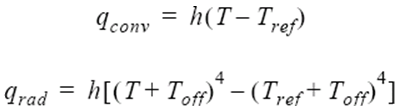where h is the value of the element boundary condition; T is the temperature; Tref is the reference temperature, given by reference_temperature; and Toff is the offset to convert to an absolute temperature, given by the absolute_temperature_offset parameter of the EQUATION command.

The turbulence_wall_type parameter determines the type of turbulence wall modeling: low Reynolds number, wall function, or wall function based on average field variables. The wall function may be modified by specifying an average wall roughness height, given by roughness_height. See the type and roughness_height parameters of the TURBULENCE_WALL command for details. An example for a wall with an imposed heat flux is given above.

The velocity boundary condition for a wall type may be specified in six different ways, as determined by wall_velocity_type. A value of match_mesh_velocity is the default and requires no further data. If the problem has a deforming mesh, that is, mesh is set to arbitrary_lagrangian_eulerian or specified in the EQUATION command, and mesh_displacement_type is set to guide_surface or flexible_body, see below, or mesh_motion is active, then the velocity is set equal to the velocity of the mesh. Otherwise, the wall velocity type reverts to zero, which sets the velocity to zero in the given reference frame. The other four wall velocity types allow the wall velocity to be given in different local coordinate systems. A wall velocity type of cartesian uses x_velocity, y_velocity, and z_velocity to impose boundary conditions in the global coordinate system, for example,
SIMPLE_BOUNDARY_CONDITION( "heated wall" ) {
...
type               = wall
wall_velocity_type = cartesian
x_velocity         = 1
y_velocity         = 0
z_velocity         = 0
...
}
A wall velocity type of cylindrical sets boundary conditions on the axial, radial, and tangential components of the velocity in a cylindrical coordinate system:
SIMPLE_BOUNDARY_CONDITION( "heated wall" ) {
type                = wall
...
wall_velocity_type  = cylindrical
cylinder_axis       = {0, 0, 0;
0, 1, 0}
axial_velocity      = 1
tangential_velocity = 0
...
}

where the axial direction is from the first to the second point in cylinder_axis, the radial direction is the outward normal from this line segment to the given node, and the tangential direction is determined by the right hand rule from the axial direction to the radial direction.

A wall velocity type of spherical imposes a given boundary condition on the radial component of the velocity in a spherical coordinate system and zero boundary conditions on the other two components:
SIMPLE_BOUNDARY_CONDITION( "heated wall" ) {
type               = wall
...
wall_velocity_type = spherical
sphere_center      = {0, 0, 0}
...
}

where the radial direction is outward from sphere_center to the given node.

A wall velocity type of normal imposes a given boundary condition on the component of the velocity normal to the surface and zero boundary conditions on the other two components:
SIMPLE_BOUNDARY_CONDITION( "heated wall" ) {
type               = wall
...
wall_velocity_type = normal
normal_velocity    = 1
...
}

where the normal direction is taken as positive inward towards the fluid domain.

The boundary conditions on the last four wall velocity types may be scaled by their corresponding multiplier functions, given by x_velocity_multiplier_function through normal_velocity_multiplier_function.

A simple boundary condition of type auto_wall simplifies the set-up of CFD models containing complex internal boundaries. All internal surfaces except for inflow, outflow, symmetry, slip and far-field, are processed automatically by AcuPrep when type = auto_wall. AcuPrep processes the auto_wall command by first identifying whether the surface is internal or external and then constructing a table of information regarding the parent volume set of each surface set. Once this information is obtained, the surface sets are prepared for the supported scenarios. When auto_wall is used, reference_frame_type and mesh_displacement_type will be set to be inherited. When interfaces are located within the CFD domain for mesh motion applications, auto_wall will automatically split interface surfaces, create the interface surface mechanism with gap and gap_factor appropriately and assign the boundary conditions. The following is an example of auto_wall input:
SIMPLE_BOUNDARY_CONDITION("auto wall"){
...
type = auto_wall
temperature_type = value
temperature = 300
turbulence_wall_type = wall_function
gap = 0.0
gap_factor = 0.0
....
}

The naming convention for the automatic generated surface outputs are as follows:

AUTO elelemtSetName1 wall

AUTO elelemtSetName1 interface

AUTO elelemtSetName1 internal

AUTO elelemtSetName2 internal

AUTO elelemtSetName2 wall

The scenarios of auto_wall are summarized in the following table:
Table 1.
Scenarios of auto_wall Examples
Solid-Solid, Same Motion Electronics cooling assembly with board+components attached.
Solid-Solid, Different Motion Spinning solid cylinder embedded within a solid box.
Fluid-Solid, Same Motion Conjugate heat transfer in a pipe (fluid/solid stationary or moving at same rate).
Fluid-Solid, Different Motion Solid wheel (smooth tread) rotating in a stationary fluid volume.
Fluid-Solid, Fluid Reference Frame Conjugate heat transfer analysis of spinning pipe. Solid exterior of pipe modeled using mesh motion, fluid interior of pipe modeled using a reference frame.
Fluid-Fluid, Same Motion Internal surface in a pipe that is created for output/visualization purposes only.
Fluid-Fluid, Different Motion Blower example with rotating impeller region and stationary outer region.
Solid-Void Conjugate heat transfer in a pipe.
Fluid-Void, No Motion External face of pipe flow case.
Fluid-Void, Motion External face of pipe flow where the pipe is retained via MRF or mesh motion.
A simple boundary condition of type inflow allows the specification of several different kinds of common inflow boundary conditions. The particular kind is specified by inflow_type. For all inflow types, nodal boundary conditions are imposed as mentioned above. A velocity inflow type imposes nodal boundary conditions on the velocity, temperature, eddy viscosity, and all species variables. No element boundary conditions are imposed. The boundary conditions on the scalar variables are specified by the temperature parameters, plus an optional scaling by the corresponding temperature_multiplier_function parameters. Boundary conditions on the velocity may be specified in different coordinate systems, as determined by inflow_velocity_type. Values of cartesian, cylindrical, spherical, and normal are supported. These are used in the same way as for wall_velocity_type, see above. Note that normal_velocity needs to be positive for flow into the fluid domain when normal is used. Defaults are used for unspecified variables. For example, a constant-flow inlet with a time-varying temperature may be specified by:
SIMPLE_BOUNDARY_CONDITION( "inlet condition" ) {
...
type                            = inflow
inflow_type                     = velocity
inflow_velocity_type            = cartesian
x_velocity                      = 1
eddy_viscosity                  = 1.e-03
temperature                     = 300
temperature_multiplier_function = "time varying"
}
MULTIPLIER_FUNCTION( "time varying" ) {
type                            = piecewise_linear
curve_fit_values                = { 0, 1.0 ;
10, 1.0 ;
20, 1.1 ;
40, 1.2 ;
80, 1.5 ; }
curve_fit_variable              = time
}
Inflow types of pressure and stagnation_pressure impose element boundary conditions on the pressure and stagnation pressure, respectively. The values used are given by the pressure and stagnation_pressure parameters, scaled by their respective multiplier function parameter stagnation_pressure_multiplier_function scales only the pressure part of the stagnation pressure. No boundary condition is imposed on any component of the velocity. The eddy viscosity as well as temperature and all species variables are specified, as in the velocity inflow type described above. Since it tends to be more stable, stagnation_pressure is preferred over pressure, as opposed to outlets where specifying the pressure is more stable. For example,
SIMPLE_BOUNDARY_CONDITION( "pressure inlet" ) {
...
type                = inflow
inflow_type         = stagnation_pressure
stagnation_pressure = 1000
eddy_viscosity      = 1.e-03
temperature         = 300
}
An inflow type of mass_flux creates a normal velocity profile across the inflow surface such that the integrated mass flux is equal to mass_flux, scaled by mass_flux_multiplier_function. The velocity profile is based on either laminar or turbulent fully-developed arbitrary cross section pipe flow, depending on the equations specified. For turbulent flows, an eddy viscosity profile based on the same fully-developed flow is also imposed. Surface nodes with zero velocity, as imposed by other boundary conditions, are considered to be the walls of the inflow surface and as such are used to compute the profile. The normal velocity profile and the eddy viscosity profile are imposed as nodal boundary conditions. The two tangential velocity components are set to zero. A flow_rate inflow type is the same as mass_flux, except the parameters flow_rate and flow_rate_multiplier_function are used, and the quantity specified is the integrated volume flux. An average_velocity inflow type is also the same as mass_flux, except the parameters average_velocity and average_velocity_multiplier_function are used, and the quantity specified is the average normal velocity. For mass_flux (mass flow rate), flow_rate (volumetric flow rate), and average_velocity inflow types the inflow surface should be planar or close to it, otherwise the meaning of normal velocity becomes vague and the results unpredictable. The temperature and all species variables are specified, as in the velocity inflow type described above. For example,
SIMPLE_BOUNDARY_CONDITION( "profiled inlet" ) {
...
type          = inflow
inflow_type   = mass_flux
mass_flux     = 25
temperature   = 300
}
For a supersonic inflow boundary condition, you need to specify all three variables. The simplest choice is primitive variables: velocity, pressure and temperature. Another choice might be: Mach number, pressure and temperature. In order to specify these conditions, the following two commands are introduced:
SIMPLE_BOUNDARY_CONDITION("superSonicInlet") {
type		= inflow
inflow_type	= velocity_pressure_temperature

or
inflow_type	= mach_pressure_temperature
}

When the specified inflow condition is subsonic, pressure is ignored, and only velocity or Mach number and temperature are used as the boundary conditions. If Mach < 1, velocity is computed by the given Mach number and temperature, while pressure is ignored. If Mach > 1, velocity is computed by the given Mach number and temperature, while pressure and temperature are fixed.

Note that both inflow_type = velocity_pressure_temperature and mach_pressure_temperature commands are only available for flow = compressible_navier_stokes.

A type outflow simple boundary condition normally, see below for the exception, imposes nodal boundary conditions on just the mesh displacement variables, see above. Element boundary conditions of type free are imposed on the tangential traction, turbulence flux, heat flux, and all the species fluxes. An element boundary condition of type outflow is imposed on the mass flux. A pressure element boundary condition is specified by the pressure parameter, plus an optional scaling by the pressure_multiplier_function parameter. For example, a typical outlet can be specified by:
SIMPLE_BOUNDARY_CONDITION( "outlet" ) {
...
type     = outflow
pressure = 0
}
Pressure loss for inflow, outflow, and far_field types may be modeled through the pressure_loss_factor parameter. The following term is added to the pressure element boundary condition, if one exists:(2)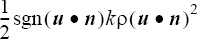where k is given by pressure_loss_factor; ρ is the density; u is the velocity; n is the outward-pointing normal to the surface; and sgnun is -1 for inflow boundaries and +1 for outflow boundaries. If pressure_multiplier_function is given, it is applied to the pressure variable before this term is added. The pressure loss factor may be scaled with pressure_loss_factor_multiplier_function. For example,
SIMPLE_BOUNDARY_CONDITION( "outflow" ) {
...
type                                     = outflow
pressure                                 = 0
pressure_loss_factor                     = 100
pressure_loss_factor_multiplier_function = "pLoss_TMF"
...
}
MULTIPLIER_FUNCTION( "pLoss_TMF" ) {
type                                    = piecewise_linear
curve_fit_values                        = { 1  , 1 ;
10 , 0 }
curve_fit_variable                      = time_step
}
The hydrostatic pressure term may be added to pressure and stagnation pressure element boundary conditions by setting hydrostatic_pressure=on. This term is given by(3)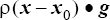where ρ is the density; x is the current coordinate vector; x0 is any coordinate vector where the hydrostatic pressure is zero, given by hydrostatic_pressure_origin; and g is the gravity vector given by body_force in the parent ELEMENT_SET command. If there are no pressure or stagnation pressure element boundary conditions, then hydrostatic_pressure has no effect. If pressure_multiplier_function or stagnation_pressure_multiplier_function is given, it is applied before this term is added. If gravitational force is modeled using the GRAVITY command, then hydrostatic_pressure=on should be set for most boundaries where pressure or stagnation pressure boundary conditions are active in order to properly account for the hydrostatic pressure. This is most commonly needed for outflow boundaries. For free surface boundaries hydrostatic_pressure is normally set to off in order to ensure that the imposed pressure is constant along the entire surface. If this pressure or the nominal height of the free surface is nonzero, then hydrostatic_pressure_origin may be adjusted for any other boundary so that its pressure boundary condition evaluated at the nominal surface height equals the pressure imposed on the free surface. That is,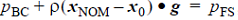, where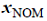is a point on the nominal free surface,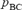is the given pressure on a surface with hydrostatic_pressure=on, and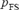is the given pressure on the free surface. For the following example, assume the nominal free surface height is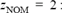GRAVITY( "gravity" ) {
type                        = constant
gravity                     = { 0, 0, -9.81 }
}
BODY_FORCE( "body force" ) {
gravity                     = "gravity"
...
}
ELEMENT_SET( "flow elements" ) {
body_force                  = "body force"
...
}
SIMPLE_BOUNDARY_CONDITION( "free surface" ) {
type                        = free_surface
...
pressure                    = 10
hydrostatic_pressure        = off
}
SIMPLE_BOUNDARY_CONDITION( "outlet" ) {
type                        = outflow
...
pressure                    = 10
hydrostatic_pressure        = on
hydrostatic_pressure_origin = { 0, 0, 2 }
}

Reflections of waves from inflow and outflow boundaries can be reduced by setting non_reflecting_factor to a positive value. This parameter applies to inflow types with inflow_type = mass_flux and to outflow types. For inflows only the mass flux element boundary condition is modified and for outflows only the pressure element boundary condition is modified. It is ignored for all other cases. Also, there is no effect for the constant density model unless a positive isothermal_compressibility value is specified.

Setting non_reflecting_factor = 0 has no effect; the given element boundary condition is imposed. Using a value of one gives the full effect; waves pass through the boundary without reflection but at the cost of the variable drifting away from its specified value. Other values yield a blend between these effects. Values greater than one for non_reflecting_factor may lead to instabilities for the mass flux condition. If running average fields are defined, that is, running_average = on in the EQUATION command, then an enhanced non-reflecting boundary condition is used, leading to less reflection for the same value of non_reflecting_factor. The non_reflecting_bc_running_average_field needs to be turned on for this option. Note that when flow = compressible_navier_stokes, the running_average option does not need to be turned on; the running average files are automatically used in non- reflecting boundary conditions.

A type slip simple boundary condition imposes a zero nodal boundary condition on the normal velocity in the given reference frame. An element boundary condition of type free is imposed on the pressure, which is important for curved surfaces. A plane of symmetry can also be specified with this type.

A type symmetry simple boundary condition is mathematically equivalent to type slip. The difference is that since a plane of symmetry must be flat a single normal is computed for the whole surface. The geometry of the given surface is checked and appropriate warning or error messages are issued if it is not flat. It is highly recommended that symmetry be used instead of slip for all surfaces that are planes of symmetry.

A type far_field boundary condition contains both a velocity-based inflow condition and a pressure-based outflow condition. At each point on the boundary the angle between the outward normal to the surface and the user-specified velocity is used to determine whether to impose the inflow or outflow conditions. The AcuSolve input for is a combination of the inflow and outflow types:
SIMPLE_BOUNDARY_CONDITION( "far field" ) {
...
type           = far_field
x_velocity     = 1
y_velocity     = 0
z_velocity     = 0
temperature    = 0
eddy_viscosity = 1.e-3
pressure       = 0
}
Sometimes there is "back flow" over a portion of an outflow or far field boundary where the velocity is into the fluid domain. This may lead to instability of the temperature, species, viscoelastic stress, kinetic energy, eddy frequency, and eddy viscosity variables. Enabling back_flow_conditions allows nodal boundary conditions to be specified for these variables only on nodes where there is flow re-entering the domain. The temperature_back_flow_type, species_1_back_flow_type ,..., species_9_back_flow_type, viscoelastic_xx_stress_back_flow_type, ..., viscoelastic_zx_stress_back_flow_type, kinetic_energy_back_flow_type, eddy_frequency_back_flow_type, and eddy_viscosity_back_flow_type parameters specify how the constant nodal boundary conditions are to be calculated. A back flow type of value uses nodal boundary conditions specified by the temperature, species_1,..., species_9, viscoelastic_xx_stress, ..., viscoelastic_zx_stress, kinetic_energy, eddy_frequency, and eddy_viscosity parameters and their corresponding multiplier function parameters. Instead of specifying a value, various solution averages may be used. A back flow type of area_average averages the solution variable over the entire outflow surface and uses the result for the nodal boundary condition. The area averaged value for the scalar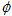is defined using the following relationship:(4)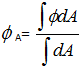where A is the area.

A back flow type of exiting_area_average averages the solution variable only over the portions of the outflow surface where flow is exiting. This is defined by the following relationship:(5)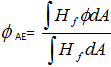where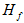is the heaviside step function defined as:(6)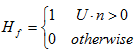A back flow type of bulk applies only to the temperature variable, and is defined as:(7)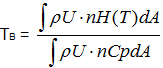where U is the velocity vector, ρ is the density, n is the surface normal vector, H is the enthalpy, Cp is the specific heat, and A is the area. A back flow type of exiting_bulk is also available for the temperature variable. When utilizing this option, the bulk temperature is averaged over only the portions of the surface where flow is re-entering the domain. The value used for the nodal constraint on temperature is computed as follows:(8)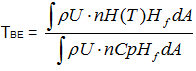Back flow types of mass_flux_average and exiting_mass_flux_average are analogous to the bulk and exiting_bulk options for temperature, but utilize mass flux averaging and apply to all scalars except temperature. In the case of mass_flux_average, mass flux weighting is used, and the value is averaged over the entire outflow surface. The relationship used to compute the scalar value becomes:(9)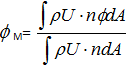A back flow type of exiting_mass_flux_average is also available for all scalars except temperature. When utilizing this option, the scalar value is mass flux averaged over only the portions of the surface where flow is re-entering the domain. The value used for the nodal constraint on the scalar is computed as follows:(10)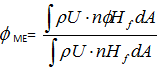If the velocity is strictly out of the fluid domain over the entire outflow boundary then back_flow_conditions has no effect. For example,
SIMPLE_BOUNDARY_CONDITION( "outlet" ) {
...
type                          = outflow
pressure                      = 0
back_flow_conditions          = on
temperature_back_flow_type    = value
temperature                   = 300.
eddy_viscosity_back_flow_type = mass_flux_average
species_1_back_flow_type      = exiting_mass_flux_average
}

A type free_surface simple boundary condition imposes nodal boundary conditions on the normal component of the mesh velocity. A pressure element boundary condition is specified by the pressure parameter, plus an optional scaling by the pressure_multiplier_function parameter.

The surface_tension_model parameter provides the surface tension information for type free_surface simple boundary conditions. This parameter specifies the user-given name of a SURFACE_TENSION_MODEL command. If this parameter is not given the surface tension defaults to zero. If it is given, then in addition a contact angle at the side walls of the free surface may be specified with the contact_angle_model parameter. This parameter specifies the user-given name of a CONTACT_ANGLE_MODEL command. If contact_angle_model is not given, the contact angle defaults to 90 degrees. See the SURFACE_TENSION_MODEL command for details.

There are two primary mechanisms by which constraints can be applied to the displacement of the mesh for moving mesh simulations. The legacy approach uses the SIMPLE_BOUNDARY_CONDITION command to assign constraints for the transport variables governing the physics of the simulation, for example velocity field, turbulence fields and species equations, as well as the movement of the mesh, while the newer approach relies on the MESH_BOUNDARY_CONDITION command to control the motion of nodes. The MESH_BOUNDARY_CONDITION command enables separation of the physics of the problem from the mesh displacement controls to provide simplified case set up in the graphical user interface. The recommended modeling guideline is to use the SIMPLE_BOUNDARY_CONDITION command to assign constraints to the physics, but use the MESH_BOUNDARY_CONDITION to assign constraints to the motion of the nodes. Note that the full set of mesh motion constraints associated with the SIMPLE_BOUNDARY_CONDITION command is still supported to provide full backward compatibility with legacy input files. However, it is recommended that these constraints be migrated to the MESH_BOUNDARY_CONDITION command.

The MESH_BOUNDARY_CONDITION command centralizes the options associated with mesh movement from the SIMPLE_BOUNDARY_CONDITION, free_surface, and EXTERNAL_CODE commands. This command is intended to act as the primary mechanism for specifying control of the mesh movement. For backward compatibility, the mesh movement related options from the SIMPLE_BOUNDARY_CONDITION, free_surface, and EXTERNAL_CODE commands are still supported by internally creating a MESH_BOUNDARY_CONDITION command for every SIMPLE_BOUNDARY_CONDITION command that is present in the input file. AcuPrep internally creates a table of constraints for each element face/node on the boundaries and identifies conflicts within the mesh motion boundary conditions. These conflicts may arise due to by the combinations of MESH_BOUNDARY_CONDITION and the legacy commands that provide similar functionality. In the case of conflicting constraints that have equal precedence values, the MESH_BOUNDARY_CONDITION is given priority. If the precedence values are not equal, then the higher precedence constraint is enforced. AcuPrep also checks for compatibility of the mesh motion boundary conditions on each node with the boundary conditions applied for the physics through the SIMPLE_BOUNDARY_CONDITION command. The following table is used when checking these compatibilities:
SIMPLE_BOUNDARY_CONDITION type MESH_BOUNDARY_CONDITION type
- fixed planar_slip mesh_motion free_surface guide_surface flexible_body external_code
wall OK OK OK Error OK OK OK
inflow OK OK OK Error OK Warning Warning
outflow OK OK OK Error OK Warning Warning
slip OK OK OK Error OK OK OK
symmetry OK OK Error Error Error Warning Error
far_field OK OK OK Error OK Error Warning
free_surface Error Error Error OK Error Warning Error
The mesh displacement boundary conditions defined above for every type is zero displacement with respect to the surface; that is, mesh nodes move with the surface. This corresponds to mesh_displacement_type = fixed, the default. Other behaviors may be obtained by setting mesh_displacement_type to none, slip, guide_surface, or flexible_body. The first of these removes all mesh displacement boundary conditions from the set. The second removes these boundary conditions and replaces them with a single condition: zero normal displacement with respect to the surface. The third is similar to slip, but the mesh displacement boundary conditions are such that the nodes slip along a guide surface given by the guide_surface parameter, which specifies a user-given name of a GUIDE_SURFACE command in the input file. Refer to the GUIDE_SURFACE command for further information and an example. The last of these, mesh_displacement_type = flexible_body, is used to specify mesh motion as part of a Fluid-Structure Interaction (FSI) problem. For example,
SIMPLE_BOUNDARY_CONDITION( "flexible surface" ) {
...
type                   = wall
mesh_displacement_type = flexible_body
flexible_body          = "flexible body"
}
FLEXIBLE_BODY( "flexible body" ) {
equation              = mesh_displacement
num_modes             = 5
...
}

where the files channel.wet_surf.xmd, channel.wet_surf.ymd, and channel.wet_surf.zmd correspond to the nodal modes in the x, y, and z-directions. The format of these files the same as nodal_modes in the NODAL_BOUNDARY_CONDITION command. See also the FLEXIBLE_BODY command for details. The nodes in these files must match those referenced in surfaces. If the problem has a deforming mesh (that is, mesh is set to arbitrary_lagrangian_eulerian or specified in the EQUATION command), and mesh_displacement_type = flexible_body and wall_velocity_type = match_mesh_velocity (the default), then the given velocity conditions are ignored. Instead, the velocity is set to match the velocity of the mesh. Note that AcuPev may be used to obtain the nodal mode files.

Mesh displacement boundary conditions may also be specified with mesh_motion. The value of this parameter refers to the user-given name of a MESH_MOTION command in the input file. This command is evaluated resulting in a mesh displacement vector. The mesh_motion parameter has an effect only if there is an active mesh equation and mesh_displacement_type is set to fixed or flexible_body. The same parameter may be specified in the parent ELEMENT_SET command, but the one here takes precedence unless precedence is set to less than zero. For example,
SIMPLE_BOUNDARY_CONDITION( "moving wall" ) {
...
type                   = wall
precedence             = 1
mesh_displacement_type = fixed
mesh_motion            = "moving body"
}
MESH_MOTION( "moving body" ) {
type                   = rigid_body
...
}
A flexible body may be combined with a rotating mesh. For example, to model a deformable impeller with sliding interfaces, for example, a wind turbine, the following commands may be used:
FLEXIBLE_BODY( "BladeA" ) {
equation               = mesh_displacement
...
}
MESH_MOTION( "Rotation" ) {
type                   = rotation
...
rotation_variable      = time
}
...
mesh_displacement_type = flexible_body
mesh_motion            = "Rotation"
}

In some circumstances it is necessary to "turn off" or "deactivate" previously-defined boundary conditions. This is accomplished by setting the active flag through active_type. A value of all is the default and means that all surfaces in the set are active (active flag = 1) and will have the boundary conditions described above imposed on them. A value of none means that no surface is active (active flag = 0).

A no_interface active type is used to automatically turn on and off boundary conditions in the presence of interfaces. Boundary conditions are applied (active flag = 1) if the surface does not belong to an INTERFACE_SURFACE set or if it does not find a contact surface of the appropriate medium. That is, each surface and boundary condition will fall into one of five possible cases:
• The surface is not in any INTERFACE_SURFACE set; active flag = 1.
• The surface is in an INTERFACE_SURFACE set, but it does not find a contact surface; active flag = 1.
• The surface finds a contact surface of fluid medium; active flag = 0.
• The surface finds a contact surface of solid/shell medium, and the boundary condition variable is defined on the solid/shell side, currently only temperature and the heat flux variables,; active flag = 0.
• The surface finds a contact surface of solid/shell medium, and the boundary condition variable is not defined on the solid/shell side, that is, it is not temperature or one of the heat flux variables,; active flag = 1.
Turbulence inflow quantities are specified according to the turbulence_input_type and turbulence model dependent properties. Legacy usage for direct specification of the eddy_viscosity variable for the spalart_allmaras based turbulence models or kinetic_energy and eddy_frequency for the sst and k_omega based turbulence models is accomplished with turbulence_input_type set to direct. As follows:
SIMPLE_BOUNDARY_CONDITION( "far field" ) {
...
type                    = far_field
x_velocity              = 1
y_velocity              = 0
z_velocity              = 0
turbulence_input_type   = direct
eddy_viscosity          = 1.e-3
}
SIMPLE_BOUNDARY_CONDITION( "inflow" ) {
...
type                   = inflow
inflow_type            = velocity
inflow_velocity_type   = normal
normal_velocity        = 31.1# m/sec
turbulence_input_type  = direct
kinetic_energy         = 0.9067 # m2/sec2
eddy_frequency         = 2.55E+5
}
The following input scenarios are also supported for turbulence_input_type = direct:
• turbulence_input_type = auto, used to automatically assign turbulent state based on selected turbulence equation, turbulence_intensity_type and turbulence_flow_type. Valid for all turbulence models.
• turbulence_input_type = intensity_length_scale, where the turbulent state at the inflow is approximated by the turbulence_intensity_type and length_scale. Valid for all turbulence models.
• turbulence_input_type = intensity_viscosity_ratio, where the turbulent state at the inflow is approximated by the turbulence_intensity_type and viscosity_ratio. Valid for all two-equation turbulence models.
• turbulence_input_type = viscosity_ratio, where the turbulent state at the inflow is approximated by the viscosity_ratio. Valid only for the spalart_allmaras based turbulence models.
The following relationships are used to compute the turbulent quantities for each turbulence model:(11)Spalart Allmaras turbulent eddy viscosity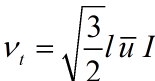(12)SST, k-ω, Standard k-ε, Realizable k-ε, RNG k-ε turbulent kinetic energy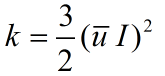(13)SST, k-ω eddy frequency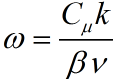or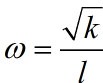(14)Standard k-ε, Realizable k-ε, RNG k-ε dissipation rate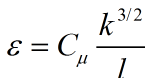or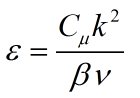Where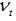is the turbulent kinematic viscosity, l is the turbulence length scale, I is the turbulence intensity,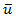is the velocity scale, k is the turbulent kinetic energy,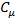=0.09, β is the ratio of turbulent to molecular viscosity, and ε is the dissipation rate. Given these relations,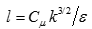for the standard, RNG, realizable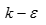models, while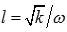for the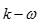and SST models. The following example illustrates the turbulence specifications when a spalart_allmaras based turbulence models is active:
SIMPLE_BOUNDARY_CONDITION( "farfield" ) {
...
type                      = far_field
x_velocity                = 14.5 # m/sec
turbulence_input_type     = auto
turbulence_flow_type      = external
turbulence_intensity_type = auto
}
The following example illustrates the turbulence specifications for turbulence_flow_type = internal when a spalart_allmaras based turbulence model is active, using turbulence_input_type = intensity_length_scale:
SIMPLE_BOUNDARY_CONDITION( "internal_inflow" ) {
...
type                       = inflow
inflow_type                = velocity
inflow_velocity_type       = cartesian
x_velocity                 = 14.5 # m/sec
turbulence_input_type      = intensity_length_scale
turbulence_flow_type       = internal
turbulence_intensity_type  = auto
turbulence_length_scale    = 0.1 #m
}
The following example illustrates the turbulence specifications when one of the two-equation based turbulence models is active, using turbulence_input_type = intensity_viscosity_ratio:
SIMPLE_BOUNDARY_CONDITION( "two_equation_farfield" ) {
...
type = far_field
x_velocity                    = 0.999847 # m/sec
y_velocity                    = 0.017452 # m/sec
z_velocity                    = 0.0           # m/sec
pressure                      = 0.0           # N/m2
turbulence_input_type         = intensity_viscosity_ratio
turbulence_intensity_type     = value
percent_turbulence_intensity  = 0.025
turbulence_viscosity_ratio    = 3.0
}
The following example illustrates the turbulence specifications when the Spalart Allmaras based turbulence models are active, using turbulence_input_type = viscosity_ratio:
SIMPLE_BOUNDARY_CONDITION( "one_equation_farfield" ) {
...
type                       = far_field
x_velocity                 = 14.5 # m/sec
turbulence_input_type      = viscosity_ratio
turbulence_viscosity_ratio = 3.0
}
The following example illustrates the turbulence specifications when one of the two-equation based turbulence models are active, using turbulence_input_type = auto and the turbulence_intensity_type = low:
SIMPLE_BOUNDARY_CONDITION( "two_equation_pressure" ) {
...
type                       = inflow
inflow_type                = pressure
pressure                   = 101325.0 #Pa
turbulence_input_type      = auto
turbulence_intensity_type  = low
turbulence_velocity_scale  = 2.0
}

Note that the turbulence specifications are turbulence model dependent and change if the simulation includes a turbulence transition model. The following tables outline the available options for each turbulence model selection with or without the inclusion of a transition model when using inflow type, with velocity inflow_type or farfield type:

 turbulence_input_type direct Input Requirements eddy_viscosity turbulence_intensity_type N/AN/A turbulence_flow_type N/A
 turbulence_input_type auto Input Requirements turbulence_flow_type turbulence_intensity_type N/A turbulence_flow_type internal external turbulence_viscosity_ratio 40.0 3.0
 turbulence_input_type intensity_length_scale Input Requirements turbulence_intensity_type length_scale turbulence_intensity_type autolow/medium/high value N/A Input Requirements turbulence_flow_typeturbulence_flow_type percent_turbulence_intensity length_scale turbulence_flow_type internal external internal external N/A percent_turbulence_intensity 4.0 0.1 1.0/4.0/10.0 0.1/0.5/1.0 As specified
 turbulence_input_type viscosity_ratio Input Requirements turbulence_viscosity_ratio turbulence_viscosity_ratio As specified
 Input Requirements turbulence_intensity_type turbulence_intensity_type autolow/medium/high value percent_turbulence_intensity 0.1 0.1/0.5/1.0 As specified
 turbulence_input_type direct Input Requirements kinetic_energyeddy_frequency dissipation_rate turbulence_intensity_type N/A turbulence_flow_type N/A
 turbulence_input_type auto Input Requirements turbulence_intensity_type turbulence_flow_type turbulence_intensity_type autolow/medium/high value Input Requirements turbulence_flow_typeturbulence_flow_type percent_turbulence_intensity turbulence_flow_type internal external internal external N/A percent_turbulence_intensity 4.0 0.5 1.0/4.0/10.0 0.1/0.5/1.0 As specified
 turbulence_input_type intensity_length_scale Input Requirements turbulence_intensity_type length_scale turbulence_intensity_type autolow/medium/high value Input Requirements turbulence_flow_type turbulence_flow_type percent_turbulence_intensity length_scale turbulence_flow_type internal external internal external N/A percent_turbulence_intensity 4.0 0.5 1.0/4.0/10.0 0.1/0.5/1.0 As specified
 turbulence_intensity_type intensity_viscosity_ratio Input Requirements turbulence_intensity_type viscosity_ratio turbulence_intensity_type autolow/medium/high value Input Requirements turbulence_flow_type turbulence_flow_type percent_turbulence_intensity turbulence_flow_type internal external internal external N/A percent_turbulence_intensity 4.0 0.5 1.0/4.0/10.0 0.1/0.5/1.0 As specified

## SST-Gamma/Gamma-Re-Theta

 turbulence_input_type direct Input Requirements kinetic_energyeddy_frequency dissipation rate turbulence_intensity_type N/A turbulence_flow_type N/A
 turbulence_intensity_type intensity_viscosity_ratio Input Requirements turbulence_intensity_type viscosity_ratio turbulence_intensity_type autolow/medium/high value Input Requirements turbulence_flow_type turbulence_flow_type percent_turbulence_intensity viscosity_ratio turbulence_flow_type internal external internal external N/A percent_turbulence_intensity 4.0 0.5 1.0/4.0/10.0 0.1/0.5/1.0 As specified
 turbulence_input_type intensity_length_scale Input Requirements turbulence_intensity_type length_scale turbulence_intensity_type autolow/medium/high value Input Requirements turbulence_flow_typeturbulence_flow_type percent_turbulence_intensity length_scale turbulence_flow_type internal external internal external N/A percent_turbulence_intensity 4.0 0.5 1.0/4.0/10.0 0.1/0.5/1.0 As specified
 turbulence_intensity_type intensity_viscosity_ratio Input Requirements turbulence_intensity_type viscosity_ratio turbulence_intensity_type autolow/medium/high value Input Requirements turbulence_flow_type turbulence_flow_type percent_turbulence_intensity viscosity_ratio turbulence_flow_type internal external internal external N/A percent_turbulence_intensity 4.0 0.5 1.0/4.0/10.0 0.1/0.5/1.0 As specified

## Atmospheric Boundary Layer Inflow

When the inflow type is set to atmospheric, inlet velocity profile and turbulence inflow quantities are generated automatically. Inlet velocity is prescribed via log law while inlet turbulence properties are computed according to the chosen turbulence model. Necessary inputs are surface roughness and reference velocity. Below are two examples of how to set inflow atmospheric boundary layer:
SIMPLE_BOUNDARY_CONDITION( "abl_inflow" ) {
...
type                                       = inflow
inflow_type                                = atmospheric
atmospheric_roughness_type                 = user_value
atmospheric_ground_roughness               = 0.001
atmospheric_reference_velocity_type        = friction_velocity
atmospheric_friction_velocity              = 1.0
}
SIMPLE_BOUNDARY_CONDITION( "abl_inflow" ) {
...
type                                      = inflow
inflow_type                               = atmospheric
atmospheric_roughness_type                = suburb
atmospheric_reference_velocity_type       = sample_height_velocity
atmospheric_sample_velocity               = 5.0
atmospheric_sample_height                 = 10.0
}

Corresponding velocity and turbulence boundary conditions at inflow will be generated automatically. Note that there should be an empirical ratio (30) between inflow roughness and standard wall roughness. For example, if atmospheric boundary layer inflow has a roughness of 0.001m, on the ground the standard turbulence wall function should have a roughness of 0.03m.